﻿ Theory of Everything

### Theory of Everything

Zhiliang Cao, Henry Gu Cao, Wenan Qiang

## Theory of Everything

Zhiliang Cao1, 2,, Henry Gu Cao3, Wenan Qiang4

1Wayne State University, 42 W Warren Ave, Detroit

2Shanghai Jiaotong University, Shanghai, China

3Northwestern University, Evanston, IL 60208, USA

4Northwestern University, 303 E Superior, Chicago IL 60611

### Abstract

A theory of everything (ToE) or final theory, ultimate theory, or master theory is a hypothetical single, all-encompassing, coherent theoretical framework of physics that fully explains and links together all physical aspects of the universe. As Newton, Einstein, and the Standard Model failed to provide such framework, Unified Field Theory (UFT) is the best framework so far. UFT gave Physics a new definition: “A natural science that involves the study of motion of space-time-energy-force to explain and predict the motion, interaction and configuration of matter.” The Torque Grid is the fundamental unit of universe. It is driven from gravity forces as result of space-time-energy-force unification. UFT deduces major Physics theories in no time. UFT unifies four major forces by resonance conditions with help of an arbitrary 3D prime wave model in which the twist/stretch ratio is 137. The resonance condition (distortion equals original size) of the Gravity force decides the size of universe and UFT concludes that the Grand universe is hierarchical. UFT builds foundation of Chemistry and Material Science with the help of predicted octahedron proton topology: proton/neutron pilings form nuclei; octahedron proton and electron interactions create cubic atoms; electron orbit overlapping decides crystal structure; electron and proton Torque and asymmetrical electron collision twist DNA. There are many UFT related papers we previously published. This paper provides main ideas of UFT papers in hopping that scientists can understand UFT better. This paper will further remove the doubts scientists have regarding the merits of the UFT theory as Theory of Everything (ToE), the final theory of the Physics.

• Zhiliang Cao, Henry Gu Cao, Wenan Qiang. Theory of Everything. Frontiers of Astronomy Astrophysics and Cosmology. Vol. 1, No. 1, 2015, pp 31-36. http://pubs.sciepub.com/faac/1/1/4
• Cao, Zhiliang, Henry Gu Cao, and Wenan Qiang. "Theory of Everything." Frontiers of Astronomy Astrophysics and Cosmology 1.1 (2015): 31-36.
• Cao, Z. , Cao, H. G. , & Qiang, W. (2015). Theory of Everything. Frontiers of Astronomy Astrophysics and Cosmology, 1(1), 31-36.
• Cao, Zhiliang, Henry Gu Cao, and Wenan Qiang. "Theory of Everything." Frontiers of Astronomy Astrophysics and Cosmology 1, no. 1 (2015): 31-36.

 Import into BibTeX Import into EndNote Import into RefMan Import into RefWorks

12345
Prev Next

### 1. Introduction

Which theory provides the foundation of physics, the Theory of Relativity, the Big-Bang, or the Standard Model? None of these existing theories can truly serve as the foundation of physics, because they cannot answer the fundamental questions, such as why positive and negative charges exist, why quantum numbers exist, and why electron has mass and never decays. In fact, the fundamental questions are ignored because they are simply too hard to answer. As the result, questionable theories are accepted as laws of physics. In order to correct the faulty theories and provide a real foundation, UFT [1-9] answers the fundamental questions. It starts from space-time-energy-force, the common root for everything, conceptual or physical.

Physics theories have become increasingly complex. The complexities give impression that physics is making good progress. These theories appeal to many people who enjoy progress. At the mean while, physics is moving side way from the true foundation, the theory that has a simple and clear starting point of space-time-energy-force.

The proposed Theory of Everything (ToE) is the all-encompassing of the theory built on the foundation of space, time, energy and force to explain and predict the motion, interaction and configuration of matter.

### 2. Torque and 3D Movements

UFT [1-9] studies arbitrary 3D motion in space and time. There are two possible arbitrary 3D movements, S twist and Z twist while straight line and circular movements are a 1D movement and a 2D movement respectively. Only the following twists are true 3D movements:Download asVeiw figureFigures index
Figure 1. Torques

One can choose three directions in a 3D coordinate system and use a String unit length to form a cube. A Torque Grid system emerges:Download asVeiw figureFigures index
Figure 2. Torque Grid

Figure 2 has a single Torque Grid with a single Torque cycle. To make it easier to remember the movement of the Torque String, this theory assumes that the main Torque String movements in the vacuum of the universe are right-handed. Positive charge has right-handed static Torque, while negative charge has left-handed static Torque.

A free Torque movement goes in all directions. The positive and negative charge is result of such 3D arbitrary motion:Download asVeiw figureFigures index
Figure 3. Arbitrary Torque Movement

As an energy carrying Torque movement creates a distortion of unit one, it moves in one direction and it makes its background moves backwards. The relative movement to the moving back ground is two. As interactive waves resonance with one another  and follow the Fibonacci sequence: 2, 3, 5, 8 ,…

2 is the relative distortion, 3*5 three axes energy, 8 is 8 faces of octahedron. An imaginary arbitrary Torque wave moves through each 8 points of cubic. When it comes back to the starting point, it gets 2 unit of distortion. Based on the simplified curve, the energy of the Torque curve is summation of three axes: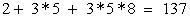(1)

It means that the Torque rotation distortion and stretch distortion has ratio of 137 when they are in resonance.

### 3. Energy and Gravity

Energy is a result of Torque Grids’ twisting distortion  when space and time are not evenly distributed. There are two types of distortions, one is twisting distortion, and another is stretching distortion.

The energy is proportional to the stretching and compression of Torque Grids . The energy differences for same matter in space lead to gravity :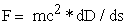(2)

Or,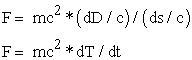(3)

Stretching and compression distortion of Torque Grids do not introduce energy in a vacuum, while the twisting distortion of Torque Grids has energy. Electron-magnetic waves are twisting distortion of the Torque Grids moving at the speed of light c.

### 4. Electron and Electromagnetic Fields

Torque Grids’ twist distortion is the basic energy form. When a Torque Grids’ twist distortion resonates with Torque Grids, the twist distortion stops light speed movements by forming a twist residue. A stable residue with the lowest possible energy has one Torque Grid twist distortion. The residue is an electron (or positron), or unit charge.

The Torque distortion extends itself outside of unit charge to form electronic fields. The movement of an electron changes the direction of electronic fields’ vector to form a magnetic field.

When the charged particle moves or circles in an inert reference, the electronic force vector starts to rotate relative to a point in the reference. The magnetic field represents the electron force vectors’ rotating movements.Download asVeiw figureFigures index
Figure 4. Curvature in An Electronic Force Line

The density of the rotating lines (assume that each line has the same strength) and the rotating speed determines the strength of the magnetic field. The next diagram studies a circling positively charged object with the following characteristics:Download asVeiw figureFigures index
Figure 5. Rotating Charge

v is the speed

Q is the amount of charge

is angular speed

B is the strength of the magnetic field

The strength of the magnetic field is:Or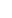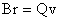Or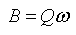Now we will use the Torque Theory to derive Ampère's circuital law equation.

In the above diagram, the magnetic field along point x at point A created by the charge at point P is: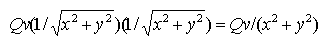Assume that the length of the wire is infinite. A unit length has Q0 charge, then v=1/t. The magnetic field will be: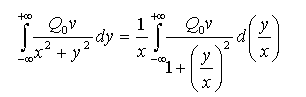Assume y/x =tan(t):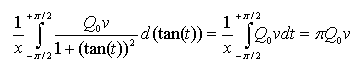Since v=1/t, and the current I in Ampere’s can be calculated with the following equation:We have: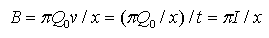Or: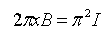The above equation can be generalized as: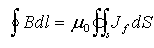(3)

The above equation is the Ampère's circuital law.

### 5. Unified Fields

5.1. Fine Structure Constant

In physics, the fine-structure constant, also known as Sommerfeld's constant, commonly denoted α, is a fundamental physical constant, namely the coupling constant characterizing the strength of the electromagnetic interaction between elementary charged particles. Being a dimensionless quantity, it has the same numerical value in all systems of units. Arnold Sommerfeld introduced the fine-structure constant in 1916.

The currently accepted value of constant α is 7.29735257×10-3 and 1/α = 137.036.

The UFT uses a mathematical formula (1) to interpret integer part of constant 137 instead of experimental driven and consider the fraction 0.036 a square vs. circular shape correction [1, 2].

The formula represents relationship between stretch distortion and twist distortion. The formula also provides a logical explanation on such constant. Amazingly, such wave formula gets a prime number as total.

Since the twist distortion is one Torque Grid size, the stretch distortion is 1/137 of Torque Grid size. The smaller Grid with 1/137 of regular Grid size is called Sub-Grid.

5.2. Strong Forces and Weak Forces

In the particle, the stretch distortion for same unit charge can be N electron distortion (1/137) as stretch distortion needs to resonance with the Sub-Grid size. When N = 137, the stretch distortion resonance with the Torque Grid and Sub-Grid, the associated force is the strong force, 137 times energy of electron.

5.3. Fraction of Weak Waves

When waves resonate with one another, the weak wave is divided further:When waves are dissonant with one another, the wave interaction are compounded:In order to properly predict the mass of the particles, the above interactions are considered to provide the calculation of the particle mass much precise as compared to standard model [10-13].

### 6. Topology of NucleiDownload asVeiw figureFigures index
Figure 6. 31Phosphorus

A nucleus is made up of protons and neutrons. Both proton and neutron share the same octahedron shape.

Octahedron protons and neutrons pile themselves up to make a nucleus. The configuration of proton/neutron octahedron pile decides the characteristics of a nucleus.

One of the important prediction of UFT is the configuration of Phosphorous.

Like other elements, Phosphorous’ nucleus collides with electron. An electron and a positron have opposite Torque movement. The figure 7 provides the annihilation process from left to right and collision of electron on a Phosphorous’ nucleus.Download asVeiw figureFigures index
Figure 7. Annihilation Process and DNA Z Twist

The two twisting unit charge become two spinning photon moving in opposite directions.

The spin motion of photon can only be meaningful when charged particle is present. As Torque is relative, the rotation direction of photon is different for different charges.

An electron can collide with proton as if annihilation occurs. When electron runs into Proton, it applies Z twists to proton; when it comes out of Proton, it applies S twist to Proton.

There are fifteen electrons for a Phosphorus atom. One of electron is unpaired. The unpaired unstable electron can twist the nucleus when nuclei are moving. The preferred twisting direction is Z twist as collision twist and electron twist (when run into the Proton) are in same Z twist direction. This can be used to explain the Z twisting force on the DNA [14-21] structure.

### 7. Hierarchical Universe

If there is a Torque Grid, then, is there anything inside the Torque Grid? Is there a boundary for our visible universe?

To answer the above questions, UFT logically induced that the universe is hierarchical . It can be infinitely large, or infinitely small in size:Download asVeiw figureFigures index
Figure 8. Universe Torque Grid Hierarchy

The universe has a simple tree structure.

A tiny Grid represents a universe. Although our visible universe is large to us, it is small Grid in a higher hierarchy universe. Everything is connected in our hierarchical unlimited universe.Download asVeiw figureFigures index
Figure 9. Universe In Your Hand

### 8. Cubic Atom and Crystal

The shape of proton has great impact on the topology of atom. Electron orbits, electron binding energy, Madelung Rules, and Zeeman splitting, are associated with proton’s octahedron shape and three nuclear structural axes. An element will be chemically stable if the outmost s and p clouds have eight electrons which make atom a symmetrical cubic.Download asVeiw figureFigures index
Figure 10. Octahedron Proton and Electron Movements

The crystal structures of the metals are related to the electron configuration and their orbit angle to the vertical line of the octahedron faces.Download asVeiw figureFigures index
Figure 11. Triangle Grids LocationDownload asVeiw figureFigures index
Figure 12. Beryllium HCP Crystal

The variation of crystal is result of eccentric cloud parings. Fortunately, octahedron proton limit the possible crystal structures.

One of interesting subjects is Lithium Phase Diagram.Download asVeiw figureFigures index
Figure 13. Lithium Phase Diagram

For Li cl16 crystal, each face of octahedron nucleus has two coupling atoms. Li cl16 has superconductivity in low temperature. Li crystal has electron coupling in Figure 14 stabilized in low temperature. The external magnetic fields can shift the electron orbit lines. New looping structure with large diameter can be formed as magnetic fields are not too strong and atom movement is relatively slow. The resonance loop of electron converses energy is the reason behind superconductivities. Hofstadter’s Butterfly [22,23-32] was observed experimentally and confirmed the possibility of looping electron, even though superconductivity looping is not observed so far.Download asVeiw figureFigures index
Figure 14. cl16 Superconducting Topology

In Figure 14, the large loop on the right hand side is essential to maintain superconductivity phase. When the wire thin enough, a large electron loop will not be possible. The experimental results tell us that if the wire is thinner than 10 nm, the cl16 superconductivity phase can hardly be observed [33-45].

### 9. Conclusion

The universe is the unification of Space, Time, Energy and Force modeled as Torque Grid. The Torque model considers Sommerfeld's constant a ratio of twist distortion and stretch distortion for a basic 3D Torque movement in resonance. The values of the four fundamental forces are determined by resonance condition of Torque movements.

The Torque energy wave resonance is also the reason behind the existence of unit charge electron and sub-atomic particles.

The predicted octahedron proton provided the insight for nuclear topologies, cubic atom, crystal structure of metals, DNA’s Z twist structure and superconductivity.

UFT also predicted that universe is composed of hierarchical Torque Grids. Everything is connected because lower universes are in your hand.

### References

  Zhiliang Cao, Henry Gu Cao. Unified Field Theory. American Journal of Modern Physics. Vol. 2, No. 6, 2013, pp. 292-298.In article View Article  Cao, Zhiliang, and Henry Gu Cao. “Unified Field Theory and the Configuration of Particles.” International Journal of Physics 1.6 (2013): 151-161.In article  Cao, Zhiliang, and Henry Gu Cao. “Unified Field Theory and the Hierarchical Universe.” International Journal of Physics 1.6 (2013): 162-170.In article  Cao, Henry Gu, and Zhiliang Cao. “Drifting Clock and Lunar Cycle.” International Journal of Physics 1.5 (2013): 121-127.In article  Cao, Zhiliang, and Henry Gu Cao. “Unified Field Theory and Topology of Nuclei.” International Journal of Physics 2, no. 1 (2014): 15-22.In article View Article  Cao, Zhiliang, and Henry Gu Cao. “Non-Scattering Photon Electron Interaction.” Physics and Materials Chemistry 1, no. 2 (2013): 9-12.In article  Cao, Zhiliang, and Henry Gu Cao. “SR Equations without Constant One-Way Speed of Light.” International Journal of Physics 1.5 (2013): 106-109.In article  Zhiliang Cao, Henry Gu Cao, Wenan Qiang, Unified Field Theory and Topology of Atom, American Journal of Modern Physics. Special Issue: Unified Field Theory: The New Physics. Vol. 4, No. 4, 2015, pp. 1-7.In article  Cao, Zhiliang, and Henry Gu Cao. “Cubic Atom and Crystal Structures.” International Journal of Physics 2, no. 6 (2014): 277-281.In article View Article  In fact, there are mathematical issues regarding quantum field theories still under debate (see e.g. Landau pole), but the predictions extracted from the Standard Model by current methods applicable to current experiments are all self-consistent. For a further discussion see e.g. Chapter 25 of R. Mann (2010). An Introduction to Particle Physics and the Standard Model. CRC Press. ISBN 978-1-4200-8298-2.In article  S.L. Glashow (1961). “Partial-symmetries of weak interactions”. Nuclear Physics 22 (4): 579–588. Bibcode: 1961NucPh..22..579G.In article  S. Weinberg (1967). “A Model of Leptons”. Physical Review Letters 19 (21): 1264–1266. Bibcode: 1967PhRvL..19.1264W.In article  A. Salam (1968). N. Svartholm, ed. “Elementary Particle Physics: Relativistic Groups and Analyticity”. Eighth Nobel Symposium. Stockholm: Almquvist and Wiksell. p. 367.In article  Kabai, Sándor (2007). “Double Helix”. The Wolfram Demonstrations Project.In article  Alberts et al. (1994). The Molecular Biology of the Cell. New York: Garland Science. ISBN 978-0-8153-4105-5.In article  “Helical repeat of DNA in solution”. PNAS 76 (1): 200–203. Bibcode: 1979PNAS...76..200W. PMC 382905. PMID 284332.In article  Pabo C, Sauer R (1984). “Protein-DNA recognition”. Annu Rev Biochem 53: 293–321. PMID 6236744.In article View Article  PubMed  James D. Watson and Francis Crick (1953). “A structure for deoxyribose nucleic acid”. Nature 171 (4356): 737–738. Bibcode: 1953Natur.171..737W. PMID 13054692.In article  Crick F, Watson JD (1954). “The Complementary Structure of Deoxyribonucleic Acid”. Proceedings of the Royal Society of London. 223, Series A: 80–96.In article  The Structure of the DNA MoleculeIn article  Wilkins MHF, Stokes AR, Wilson HR (1953). “Molecular Structure of Deoxypentose Nucleic Acids” (PDF). Nature 171 (4356): 738–740. Bibcode: 1953Natur.171.. 738W.In article  Cheng Chin, Erich J. Mueller, “Viewpoint: Looking for Hofstadter’s Butterfly in Cold Atoms,” Physics 6, 118 (2013).In article View Article  D. R. Hofstadter, “Energy Levels and Wave Functions of Bloch Electrons in Rational and Irrational Magnetic Fields,” Phys. Rev. B 14, 2239 (1976).In article View Article  M. C. Geisler, J. H. Smet, V. Umansky, K. von Klitzing, B. Naundorf, R. Ketzmerick, and H. Schweizer, “Detection of a Landau Band-Coupling-Induced Rearrangement of the Hofstadter Butterfly,” Phys. Rev. Lett. 92, 256801 (2004).In article View Article  PubMed  S. Melinte et al., “Laterally Modulated 2D Electron System in the Extreme Quantum Limit,” Phys. Rev. Lett. 92, 036802 (2004).In article View Article  PubMed  T. Feil, K. Výborný, L. Smrcka, C. Gerl, and W. Wegscheider, “Vanishing Cyclotron Gaps in a Two-Dimensional Electron System with a Strong Short-Period Modulation,” Phys. Rev. B 75, 075303 (2007).In article View Article  C. R. Dean et al. “Hofstadter’s Butterfly and the Fractal Quantum Hall Effect in Moire Superlattices,” Nature 497, 598 (2013).In article View Article  PubMed  L. A. Ponomarenko et al., “Cloning of Dirac Fermions in Graphene Superlattices,” Nature 497, 594 (2013).In article View Article  PubMed  M. Aidelsburger, M. Atala, M. Lohse, J. T. Barreiro, B. Paredes, and I. Bloch, “Realization of the Hofstadter Hamiltonian with Ultracold Atoms in Optical Lattices,” Phys. Rev. Lett. 111, 185301 (2013).In article View Article  PubMed  H. Miyake, G. A. Siviloglou, C. J. Kennedy, W. C. Burton, and W. Ketterle, “Realizing the Harper Hamiltonian with Laser-Assisted Tunneling in Optical Lattices,” Phys. Rev. Lett. 111, 185302 (2013).In article View Article  PubMed  R. E. Peierls, “On the Theory of Diamagnetism of Conduction Electrons,” Z. Phys. 80, 763 (1933).In article View Article  P. G. Harper, “Single Band Motion of Conduction Electrons in a Uniform Magnetic Field,” Proc. Phys. Soc. A 68, 874 (1955).In article View Article  D. J. Thouless, M. Kohmoto, M. P. Nightingale, and M. den Nijs, “Quantized Hall Conductance in a Two-Dimensional Periodic Potential,” Phys. Rev. Lett. 49, 405 (1982).In article View Article  Rogachev, A. and Wei, T.-C. and Pekker, D. and Bollinger, A. and Goldbart, P. and Bezryadin, A., “Magnetic-Field Enhancement of Superconductivity in Ultranarrow Wires,” Phys. Rev. Lett. 97, 13 (2006).In article View Article  Bezryadin, A.; Lau, C. N.; Tinkham, M. Nature 2000, 404, 971.In article View Article  PubMed  Lau, C. N.; Markovic, N.; Bockrath, M.; Bezryadin, A.; Tinkham, M.; Phys. ReV. Lett. 2001, 87, 217003.In article View Article  PubMed  Zgirski, M.; Riikonen, K. P.; Touboltsev, V.; Arutyunov, K.; Nano Lett. 2005, 5, 1029.In article View Article  PubMed  Tinkham, M. Introduction to SuperconductiVity, 2nd ed.; McGraw-Hill: New York, 1996.In article  Tian, M. L.; Wang, J. G.; Kurtz, J. S.; Liu, Y.; Chan, M. H. W.; Mayer, T. S.; Mallouk, T. E.; Phys. ReV. B 2005, 71, 104521.In article View Article  Altomare, F.; Chang, A. M.; Melloch, M. R.; Hong, Y. G.; Tu, C. W.; Phys. ReV. Lett. 2006, 97, 017001.In article View Article  PubMed  Xu, K.; Heath, J. R.; Nano Lett. 2008, 8, 136.In article View Article  PubMed  Hopkins, D. S.; Pekker, D.; Goldbart, P. M.; Bezryadin, A.; Science 2005, 308, 1762.In article View Article  PubMed  Makhlin, Y.; Schon, G.; Shnirman, A.; Nature 1999, 398, 305.In article View Article  Nakamura, Y.; Pashkin, Y. A.; Tsai, J. S.; Nature 1999, 398, 786.In article View Article  Mooij, J. E.; Nazarov, Y. V.; Nat. Phys. 2006, 2, 169.In article View Article